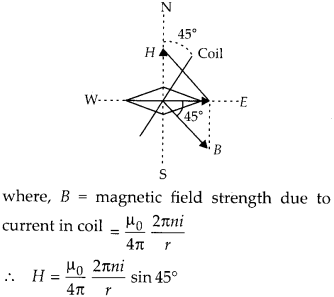Enlightened

# Question 20: NCERT Solutions for 12th Class Physics: Chapter 5-Magnetism and Matter

• 0

Question 20: NCERT Solutions for 12th Class Physics: Chapter 5-Magnetism and Matter

A compass needle free to turn in a horizontal plane is placed at the centre of a circular coil of 30 turns and radius 12 cm. The coil is in a vertical plane making an angle of 45° with the magnetic meridian when the current in the coil is 0.35 A, the needle points west to east.
(a) Determine the horizontal component of earth’s magnetic field at the location.
(b) The current in the coil is reversed and the coil is rotated about its vertical a×is by an angle of 90° in the anticlockwise sense looking from above. Predict the direction of the needle. Take the magnetic declination at the places to be zero.

Share

1. Solution:
Here, n = 30, r = 12 cm = 12 × 10-2 m. i = 0.35 A ,H=? As is clear from figure shown the needle can point west to east only when H = B sin 45°(b) When current in coil is reversed and coil is turned through 90° anticlockwise, the direction of needle will reverse (i.e. it will point from east to west). This follows from figure shown.

Check the complete chapter with solutions.

NCERT Solutions for 12th Class Physics: Chapter 5-Magnetism and Matter

• 0